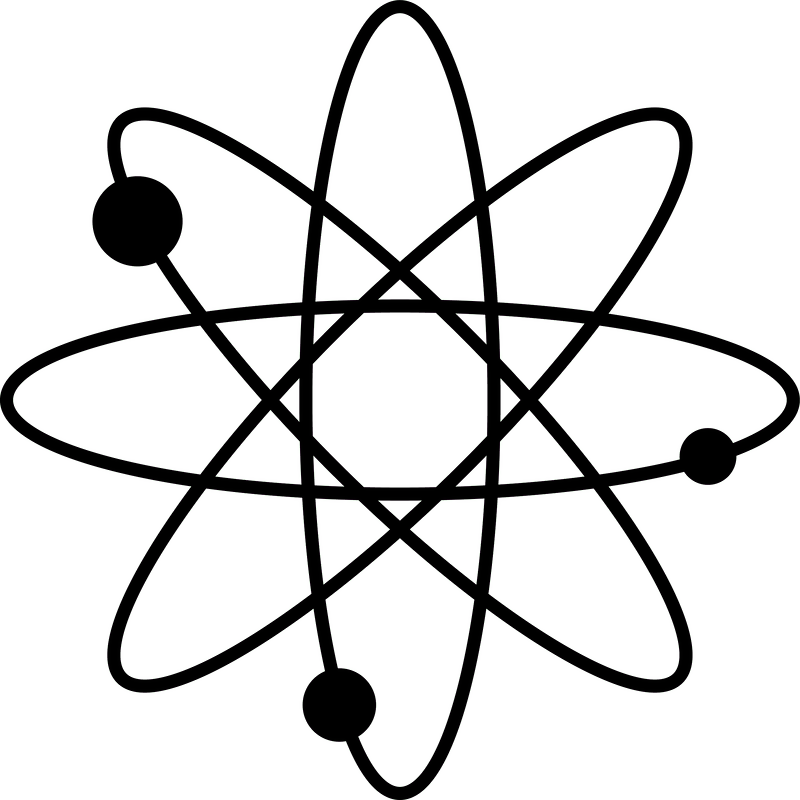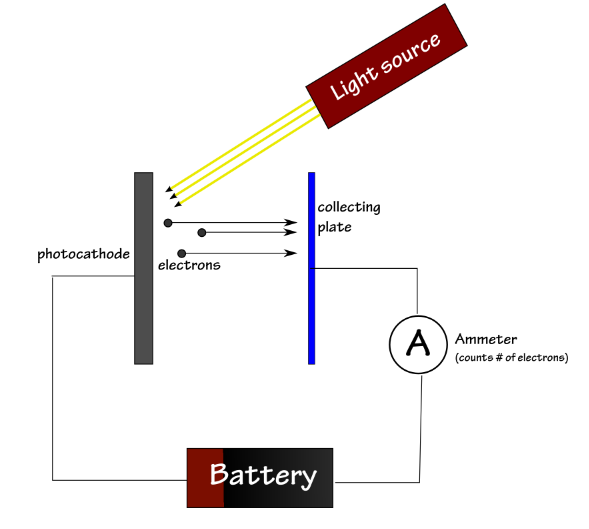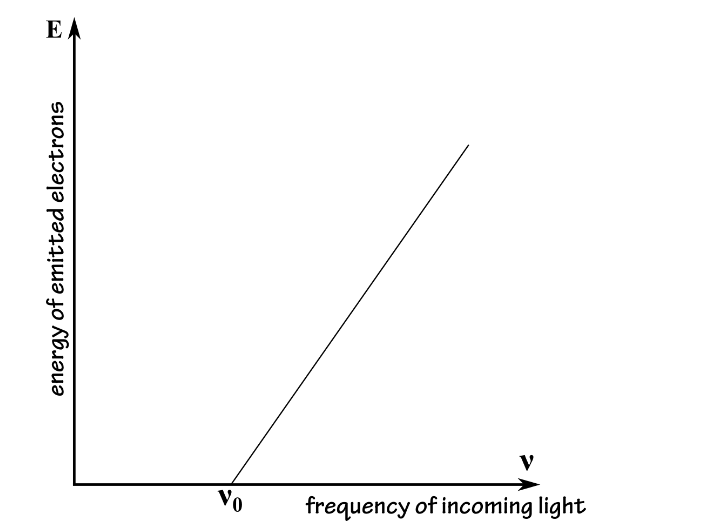###Physics Primer: The Photoelectric Experiment

The PHotoelectric Experiment

The photoelectric effect is illustrated bythe following experiment: We shine light on a metal plate, called aphotocathode (or cathode). We use a special light source for which we can varythe frequency. Above a certain frequency, the photocathode will emit electronsthat are collected by a second metal plate. We can connect the two metal platesby a wire and an ammeter to measure the intensity of the current we haveproduced. If we want to determine the kinetic energy of the emitted electronswe can also connect to the circuit a power supply that adds a voltage betweenthe two metal plates.When the electrons are emitted by thephotocathode they have a certain velocity and kinetic energy Ekinetic.When we introduce the voltage (and implicitly the electric field) the electronswill be slowed down. Above a certain voltage, the electrons would stopcompletely between the two metal plates, and they would accelerate back towardsthe photocathode. The minimum value of the voltage for which the electrons turnback corresponds to an electric energy that matches the initial kinetic energyof the electron:

Eelectric=Ekinetic

The electric energy corresponding to thevoltage is: Eelectric=eV,where e is the charge of an electron and V is the voltage.

For the purpose of this course it is notnecessary to know where this equation is coming from, but if you are wonderingabout it you should know that this relation defines the electric voltage: avoltage "is the electric energy charge difference of electric potentialenergy transported between two points" (http://en.wikipedia.org/wiki/Voltage)

Using this relation we can find the kineticvalue of the emitted electrons:

Ekinetic=eV

Using this setup, we can measure and thenplot the energy of the electrons emitted by the cathode versus the frequency ofthe light that is pointed towards the cathode. The result would look like theplot bellow:This result could not be explained by theclassical theory of light waves. According to classical physics, the energycarried by a wave of light is proportional to its intensity, so the energytransmitted to the escaping electrons would be expected to vary with theintensity of the incident light: the brighter the light, the more energetic theelectrons. Likewise, there shouldn’t have been any lower threshold frequencyneeded to eject the electrons: a bright enough light should have carried enoughenergy to free the electrons from the metal even for a low frequency. Instead,the energy carried by each electron was independent of the intensity of theincident light rays; only the number of emitted electrons depended on theintensity of light, but not their energy.

Einstein's Solution

Opposed to the other physicists whounanimously treated light as a wave, Einstein assumed that one could considerlight as being made out of discrete particles (which were later dubbed“photons”). Einstein used these particles as a "heuristic", a usefulbut provisional explanation that did not necessarily correspond to the truenature of light.

与其他一致认为光是波浪的物理学家不同，爱因斯坦认为人们可以认为光是由离散粒子（后来称为光子）制成的。爱因斯坦用这些粒子作为“启发”，一个有用但暂时的解释，并不一定符合光的本质。

How would the photoelectric effect lookfrom the point of view of the atoms in the cathode, if light behaved like aparticle? Before the light source is turned on, the electrons in the cathodeare bound to their atoms with a certain binding energy, which is acharacteristic of the specific kind of metal used in the cathode, and which wemay denote by Φ. In order to be torn free of their host atoms, the electronswould need to receive an amount of energy in excess of this binding energy. (Sofar, both the wave theory and the particle theory of light would agree withthis assumption.)

The light source is switched on. Instead ofa continuous wave of light washing over the surface of the cathode, imagine theelectrons in the cathode being hit by lots and lots of separate photons. Eachphoton would carry a definite amount of energy, given by Planck's equation:

Ephoton=hν

Each time one of the photons hits anelectron in the cathode, it would collide much as billiard balls collide,transferring its energy to the electron. If the energy of the incoming photon wasless than the electron’s binding energy, the electron, even after absorbing thephoton’s energy, would be unable to escape from its atom: no ejected electrons,and no measured current. Maybe counterintuitively, if two photons hit anelectron immediately one after the other, the energy they would transmit to theelectron will not add up. After being hit by a photon, the electron might bemore energetic for a very brief period of time, but almost immediately it willfall back in its low energy state. When the second photon hits the electron,the electron will be back in its initial state.

Think of a garbage can half-filled withping pong balls. You are trying to get some of the balls out by throwingpennies in. If you throw the pennies with insufficient energy, it doesn’tmatter how many pennies you throw, you won’t bounce a ping-pong ball out. Yetif you throw even a single penny with enough energy, you can bounce a ball outof the can. A photocathode exposed to high-frequency light works in a similarway. If the incoming photons carried more energy than the binding energy, thenafter colliding with an electron and transferring its energy, the electronwould be torn free from its atom, and would stream toward the collecting platewith a kinetic energy

Ekinetic=hν−Φ

By thinking of light as made up of theseparticle-like photons, rather than as a continuous wave, Einstein could explainall the features of the photoelectric effect: a single photon (carrying energyEphoton=hν) would be sufficient to knock out an electron, and henceproduce a current in the circuit, so long as hν>Φ. A greater intensity ofthe light source would just mean more photons, but each carrying the sameenergy hν. With more photons hitting the surface, more collisions betweenphotons and electrons would take place, resulting in more electrons beingemitted — yet each electron, having been knocked free by its collision with anincoming photon, would carry the same energy, hν−Φ. Thus, even a very intenselight would be unable to release any electrons (and hence would produce nocurrent) if the energy carried by its photons fell below the binding energy.